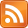SMS scnews item created by Daniel Daners at Fri 24 May 2019 1129
Type: Seminar
Modified: Fri 24 May 2019 1149; Fri 24 May 2019 1152
Distribution: World
Expiry: 27 May 2019
Calendar1: 27 May 2019 1400-1500
CalLoc1: AGR Carslaw 829
CalTitle1: Hauer: Fractional powers of monotone operators in Hilbert spaces
Auth: daners@dora.maths.usyd.edu.au

# Fractional powers of monotone operators in Hilbert spaces

### Hauer

Daniel Hauer
University of Sydney
Mon 27th May 2019, 2-3pm, Carslaw Room 829 (AGR)

## Abstract

In this talk I want to present a functional analytical framework for defining fractional powers of maximal monotone (possibly, multi-valued and nonlinear) operators in Hilbert spaces. We begin by showing that if $A$ is a maximal monotone operator on a Hilbert space $H$ with $0$ in the range $Rg\left(A\right)$ of $A$, then for every $0, the Dirichlet problem

 (${D}_{\phi }^{s}$)

associated with the Bessel-type operator ${B}_{1-2s}u:=-\frac{1-2s}{t}{u}_{t}-{u}_{tt}+Au$ is well-posed for every boundary value $\phi \in {\overline{D\left(A\right)}}^{{\text{}}_{H}}$. This enables us to investigate the Dirichlet-to-Neumann (D-t-N) operator

$\phi ↦{\Lambda }_{s}\phi :=-\underset{t\to 0+}{lim}{t}^{1-2s}{u}_{t}\left(t\right)$

on $H$ (where $u$ solves (${D}_{\phi }^{s}$)) associated with ${B}_{1-2s}$ and to define the (${L}_{s}^{2}$) fractional power ${A}^{s}$ of $A$ via the extension problem (${D}_{\phi }^{s}$). We investigate the semigroup ${\left\{{e}^{-{A}^{s}t}\right\}}_{t\ge 0}$ generated by $-{A}^{s}$ on $H$; prove comparison principles, contractivity properties of ${\left\{{e}^{-{A}^{s}t}\right\}}_{t\ge 0}$ in Orlicz spaces ${L}^{\psi }$, and show that ${A}^{s}$ admits a sub-differential structure provided $A$ has it as well.

The results extend earlier ones obtained in the case $s=1∕2$ by Brezis  [Israel J. Math. 72], Barbu [J. Fac. Sci. Univ. Tokyo Sect. IA Math.72.].

As a by-product of the theory developed in the presented work, we also obtain well-posedness of the Robin problem associated with ${B}_{1-2s}$, which might be of independent interest.

The results are joint work with the two former undergraduate student Yuhan He and Dehui Liu during summer 2018 at the University of Sydney.

For Seminar announcements you can now subscribe to theSeminar RSS feed. Check also the PDE Seminar page.

Enquiries to Daniel Hauer or Daniel Daners.

If you are registered you may mark the scnews item as read.
School members may try to .